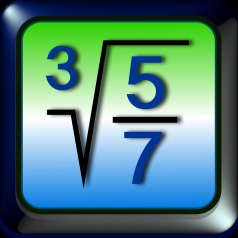iMathics

iMathics is a calculator for the daily use, for the school and university and for technical purposes

The iMathics calculator is available in the Apple App Store and is designed for the iPhone, the iPod touch and the iPad.

Differences to other calculators:

• The mathematical expressions can be entered in the same way as they are written
• By errors detailed error messages are displayed highlighting the error origin
• The results can be shown as decimal numbers, as simple or mixed fractions
• The display can be zoomed with finger pinch gestures
• The most complicated mathematical expressions can be input over a simple keyboard
• Representation of the expressions like in the mathematics books
• Mathematical correct calculus (!) with mixed fractions
• Percentage calculations